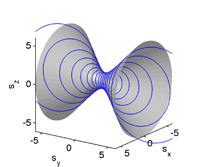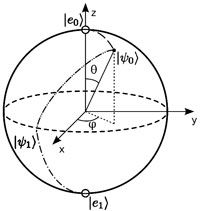May 2011
Mon Tue Wed Thu Fri Sat Sun
« Apr   Jun »
1
2345678
9101112131415
16171819202122
23242526272829
3031

## PT symmetry and Weyl asymptotics

Johannes Sjoestrand

For a class of PT-symmetric operators with small random perturbations, the eigenvalues obey Weyl asymptotics with probability close to 1. Consequently, when the principal symbol is non-real, there are many non-real eigenvalues.

http://arxiv.org/abs/1105.4746
Spectral Theory (math.SP)

## Coquaternionic quantum dynamics for two-level systems

Dorje C. Brody, Eva-Maria GraefeThe dynamical aspects of a spin-1/2 particle in Hermitian coquaternionic quantum theory is investigated. It is shown that the time evolution exhibits three different characteristics, depending on the values of the parameters of the Hamiltonian. When energy eigenvalues are real, the evolution is either isomorphic to that of a complex Hermitian theory on a spherical state space, or else it remains unitary along an open orbit on a hyperbolic state space. When energy eigenvalues form a complex conjugate pair, the orbit of the time evolution closes again even though the state space is hyperbolic.

http://arxiv.org/abs/1105.4038
Mathematical Physics (math-ph); Quantum Physics (quant-ph)

## Some Remarks on Quantum Brachistochrone

Fabio MasilloWe study some aspects of the Quantum Brachistochrone Problem. Physical realizability of the faster pseudo Hermitian version of the problem is also discussed. This analysis, applied to simple quantum gates, supports an informational interpretation of the problem that is quasi Hermitian invariant.

http://arxiv.org/abs/1105.3332
Quantum Physics (quant-ph)

## Two-parametric PT-symmetric quartic family

Alexandre Eremenko, Andrei GabrielovWe describe a parametrization of the real spectral locus of the two-parametric family of PT-symmetric quartic oscillators. For this family, we find a parameter region where all eigenvalues are real, extending the results of Dorey, Dunning, Tateo and Shin.

http://arxiv.org/abs/1105.2742
Mathematical Physics (math-ph)

## Discrete quantum square well of the first kind

Miloslav ZnojilA toy-model quantum system is proposed. At a given integer $$N$$ it is defined by the pair of $$N$$ by $$N$$ real matrices $$(H,\Theta)$$ of which the first item $$H$$ specifies an elementary, diagonalizable non-Hermitian Hamiltonian $$H \neq H^\dagger$$ with the real and explicit spectrum given by the zeros of the $$N-$$th Chebyshev polynomial of the first kind. The second item $$\Theta\neq I$$ must be (and is being) constructed as the related Hilbert-space metric which specifies the (in general, non-unique) physical inner product and which renders our toy-model Hamiltonian selfadjoint, i.e., compatible with the Dieudonne equation $$H^\dagger \Theta= \Theta\,H$$. The elements of the (in principle, complete) set of the eligible metrics are then constructed in closed band-matrix form. They vary with our choice of the $$N-$$plet of optional parameters, $$\Theta=\Theta(\vec{\kappa})>0$$ which must be (and are being) selected as lying in the positivity domain of the metric, $$\vec{\kappa} \in {\cal D}^{(physical)}$$.

http://arxiv.org/abs/1105.1863
Quantum Physics (quant-ph); High Energy Physics – Lattice (hep-lat); Mathematical Physics (math-ph)

## Momentum and Hamiltonian in Complex Action Theory

Keiichi Nagao, Holger Bech Nielsen

In the complex action theory (CAT) we explicitly examine how the momentum and Hamiltonian are defined from the Feynman path integral (FPI) point of view. In arXiv:1104.3381[quant-ph], introducing a philosophy to keep the analyticity in parameter variables of FPI and defining a modified set of complex conjugate, hermitian conjugates and bras, we have extended $$| q >$$ and $$| p >$$ to complex $$q$$ and $$p$$ so that we can deal with a complex coordinate $$q$$ and a complex momentum $$p$$. After reviewing them briefly, we describe in terms of the newly introduced devices the time development of a $$\xi$$-parametrized wave function, which is a solution to an eigenvalue problem of a momentum operator $$\hat{p}$$, in FPI with a starting Lagrangian. Solving the eigenvalue problem, we derive the momentum and Hamiltonian. Oppositely, starting from the Hamiltonian we derive the Lagrangian in FPI, and we are led to the momentum again via the saddle point for $$p$$. This study confirms that the momentum and Hamiltonian in the CAT have the same forms as those in the real action theory. We also show the third derivation of the momentum via the saddle point for $$q$$.

http://arxiv.org/abs/1105.1294
Quantum Physics (quant-ph); High Energy Physics – Theory (hep-th)